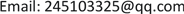——基于多元线性模型和分类研究1. 引言1.1. 课题背景

1.2. 数据简介1.2.1. 数据来源

1.2.2. 变量解释

1.2.3. 变量分类

2. 典型相关分析

\$cor

 0.8422213 0.6919939 0.4832242 0.2977800 0.1298041 0.0638123

\$xcoef

[,1] [,2] [,3] [,4] [,5] [,6]

\$ycoef

[,1] [,2][,3] [,4] [,5] [,6]

\$xcenter

3.14489e − 17 2.05928e − 17 −1.07441e − 17 1.65638e − 17 1.16394e − 17 4.09898e − 18

\$ycenter

1.99213e − 17 1.34301e − 17 −4.70054e − 181.81866e − 172.95463e − 177.16273e − 18

\$cor给出了典型相关系数，体现了各对典型变量的相关性从大到小的性质，r1 = 0.842，r2 = 0.692，因此这两组数据存在高度相关的关系，即混合类物质越多，微量的重金属元素前两对标准化的典型变量的线性组合是：

U 1 = 0.349 X 1 + 0.308 X 2 + 0.074 X 3 − 0.025 X 4 − 0.045 X 5 + 0.5322 X 6

V 1 = − 0.168 Y 1 + 0.826 Y 2 + 0.444 Y 3 − 0.298 Y 4 + 0.722 Y 5 + 0.216 Y 6

U 2 = − 0.361 X 1 − 0.085 X 2 + 0.069 X 3 + 0.224 X 4 − 0.056 X 5 + 0.263 X 6

V 2 = 0.088 Y 1 − 0.084 Y 2 − 0.248 Y 3 + 0.328 Y 4 − 0.064 Y 5 − 0.076 Y 6

3. 多元线性回归3.1. 理论基础3.1.1. 最小二乘估计

Y = β 0 + β 1 X 1 ⋯ + β p − 1 X p − 1 + e

y = ( y 1 y 2 ⋯ y n ) , β = ( β 1 β 2 ⋯ β n ) ， X = ( 1 X 11 ⋯ X 1 P 1 X 21 ⋯ X 2 P ⋯ ⋯ 1 X n 1 ⋯ X n p ) , ε = ( ε 1 ε 2 ⋯ ε n )

β的最小二乘估计 β ^ = ( X T X ) − 1 X T y ，残差向量为 ε ^ = y − X β ^ ， 的最小二乘估计为 σ ^ 2 = ε ^ T ε ^ n − p − 1 。

3.1.2. 显著性检验

1) 回归方程线性关系的检验

H 0 : β 0 = β 1 = ⋯ = β p = 0 ， H 1 : β 0 ， β 1 ， ⋯ , β p 至少有一个不为0

2) 回归系数显著性检验

H 0 : β i = 0 ， H 1 : β i ≠ 0 ( i = 1 , 2 , 3 ⋯ , p )

t i = β ^ i s β ^ i 服从t(n−p)的t分布

3.2. 模型的建立

Y = β 0 + β 1 X 1 + β 2 X 2 + β 3 X 3 + β 4 X 4 + β 5 X 5 + β 6 X 6 + β 7 X 7 + β 8 X 8 + β 9 X 9 + β 10 X 10 + β 11 X 11

Y 1 = 5.289 + 9.840 X 4 − 0.003 X 11 − 0.001 X 13

Y 2 = − 36.579 + 0.889 X 3 + 14.089 X 4 − 0.430 X 7 + 0.272 X 9

3.3. 回归诊断3.3.1. 残差和残差图

1) V a r ( e i ) = σ 2

2) C o v ( e i e j ) = 0 ，其中i ≠ j

Y ^ = X β ^ = X ( X T X ) − 1 X T Y = H Y

3.3.2. 异常点检验

D i ( σ ) = h i i 1 − h i i ⋅ ε ^ i σ ^ ( i ) 1 − h i i

D i ( σ ) > 2 p + 1 n

123 4 5 6 7 8 9 1011 12

FALSE FALSE FALSE FALSE FALSE FALSE FALSETRUE FALSE FALSE FALSE FALSE

13 14 15 1617 18 19 20 212223 24

FALSE FALSE FALSETRUE FALSE FALSE FALSE FALSE FALSE FALSE FALSE FALSE

25 2627 28 29 30 31

FALSE FALSE FALSE FALSE FALSE FALSE FALSE

Y 1 = 4.656 + 9.367 X 4 − 0.003 X 11 − 0.0007 X 13

3.3.3. 多重共线性诊断

V a r ( β ^ j ) = σ 2 ∑ x j 2 ⋅ 1 1 − R j 2 = σ 2 ∑ x j 2 = σ 2 ∑ x j 2 ⋅ V I F j

VIFj即为变量Xj的方差扩大因子， R j 2 为自变量Xj对其余自变量作回归分析的复相关系数。方差扩

vif(aa)

x4 x11 x13

1.835484 1.172172 1.619351

> sqrt(vif(aa))>2

x4 x11 x13

FALSE FALSE FALSE

4. 线性模型解释

5. 总结